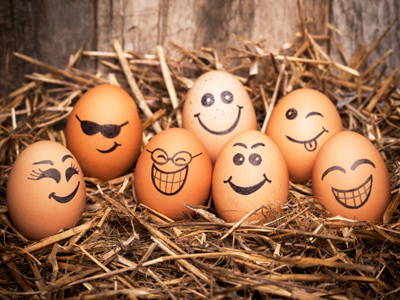Eggs normally come in 'half a dozen' boxes. Do you know how many is half a dozen?

# 12 Times Table

Learning the times tables is a vital tool when multiplying numbers. By the end of Year 2 children are often fluent in their times tables up to 10. When they enter into KS2 in Year 3 they will also be taught some higher times tables in their Maths and Numeracy lessons. By the end of KS2 they will be familiar with all of the times tables from one to twelve. This quiz will test their knowledge of the 12 times table.

Learning the 12 times table is all about multiplying numbers by 12. We only have room for 10 questions, so we have dropped the 'easy' 1 x 12 and 10 x 12. Therefore, you might find this quiz more of a challenge than the other times table quizzes!

Learning your times tables will help you whenever you need to multiply numbers in real life. See if you can get top marks - and if you don't manage it first time - give it another shot until you do!

1.
6 x 12 =
60
67
71
72
2.
12 x 12 =
114
144
156
166
The number 144 is sometimes called 'one gross'
3.
12 x 4 =
44
46
48
50
4.
12 x 11 =
132
121
111
101
If you know your 11 times table, then you should already know this one!
5.
12 x 9 =
88
111
121
108
6.
12 x 2 =
24
14
34
26
The number 12 is also called a dozen
7.
7 x 12 =
84
88
92
108
An easy way to work out this one is to do 7 x 10, then 7 x 2 and add the two totals together
8.
5 x 12 =
52
58
60
65
We could write 12 + 12 + 12 + 12 + 12 to get the same outcome, but it's much easier to use the multiplication symbol
9.
3 x 12 =
36
15
24
33
10.
12 x 8 =
80
87
93
96
You can find more about this topic by visiting BBC Bitesize - 11 and 12 times table

Author:  Sarah Garratty